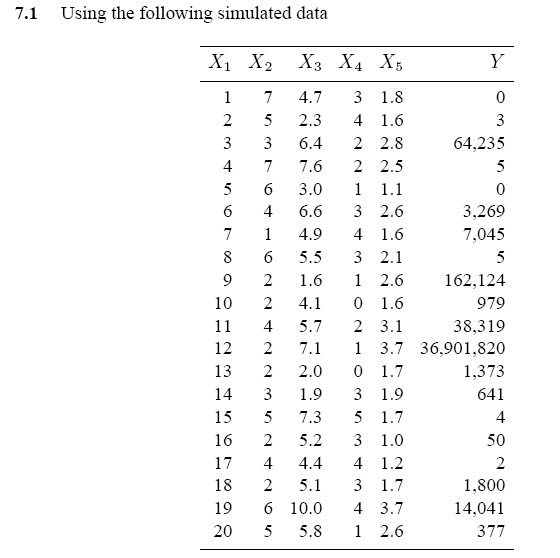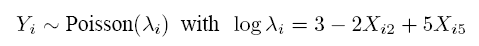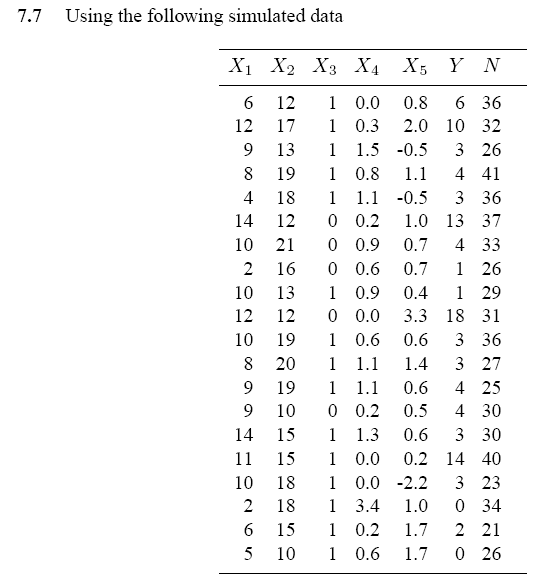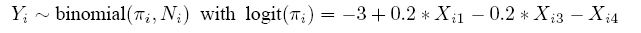## Microsoft word - assignments_2013.docx

ASSIGNMENT 2 for Marianna Marra
The dataset ear.dat is based on 214 children with acute otitis media (OME) who participated in a randomized clinical trial (Mandel et.al., 1982,Pedriatic Infectious Diseases, 1, 310-316). Each child had OME at the beginning of the study in either one (unilateral cases) or both (bilateral cases) ears. Each child was randomly assigned to receive a 14-days course of one of antibiotic, either cefaclor (CEF) or amoxicillin (AMO). The focus here is on the 203 children whose middle ear status was determined at a 14-day follow up visit. The data have the following Column Variable Format or Code ------------------------------------------------------------------------- 1-3 ID 5 Clearance by 14 days 1=yes/0=no 7 Antibiotic 1=CEF/2=AMO 9 Age 1=<2 yrs/2=2-5 yrs/3=6+ yrs 11 Ear 1=1st ear/2=2nd ear ------------------------------------------------------------------------ 1. Construct a model in WinBUGS to estimate the efficiency of the drug treatment considering also other possible risk factors. Assume two models: one with no correlation between the two ears and one by introducing correlation via random effects. What are the differences between the two models? Are random effects necessary here? 2. Perform Bayesian variable selection to estimate the covariates. Specify carefully your prior and also perform sensitivity analysis on different values of the prior variance. What is your final conclusion? 3. Interpret the results of the median probability model, maximum-a-posteriori model andASSIGNMENT 2 for Flavia Fornaciari
Implement the Bayesian variable selection methods to estimate which model and which variables should be included in the model. Be careful with the prior selection and also conduct a sensitivity analysis. Interpret the posterior results for the Median probability and the Maximum a-posteriori models (if different). Repeat the same analysis for the same for the model averaged coefficients. Compare the results with the true expression used to generate the data: ASSIGNMENT 2 for Ilaria Masiami
athens.aueb.gr/~jbn/winbugs_book/home.html). The data were compiled from a customer satisfaction survey contacted in Chios, Greece (Sarantinidis, 2003). The given dataset is only part of the original dataset. Explanation of the variables is provided in file mshop1.txt.
• Build an ANCOVA model and identify which variables influence the total money spent using Bayesian variable selection methods. Be careful with the prior specification. Conduct also sensitivity analysis on the results. • Interpret the posterior results for the Median probability and the Maximum a-posteriori • Repeat the same analysis for the same for the model averaged coefficients.ASSIGNMENT 2 for Riccardo
Implement the Bayesian variable selection methods to estimate which model and which variables should be included in the model. Be careful with the prior selection and also conduct a sensitivity analysis. Interpret the posterior results for the Median probability and the Maximum a-posteriori models (if different). Repeat the same analysis for the same for the model averaged coefficients. Compare the results with the true expression used to generate the data: